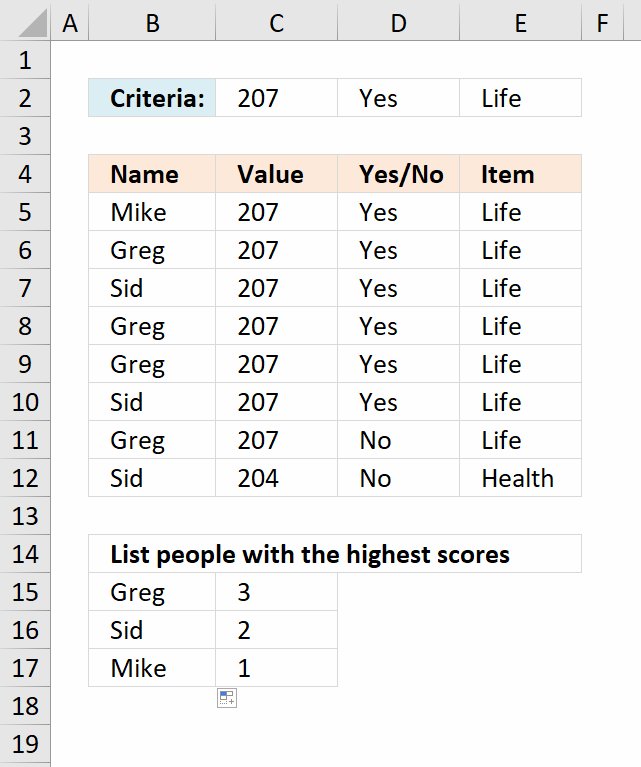Author: Oscar Cronquist Article last updated on January 05, 2019Andre asks:I am trying to list people with the highest scores based on certain criteria.

My data:

column

A B C D

Mike 207 Yes Life
Greg 207 Yes Life
Sid 207 Yes Life
Greg 207 Yes Life
Greg 207 Yes Life
Sid 207 Yes Life
Greg 207 No Life
Sid 204 No Health

criteria
countif b= 207 and column c= yes and column d= Life

and it then needs to arrange it from the highest to the lowest
(Large)

and then match it with the name eg, Greg Sid or Mike

so what I am looking for is eg.

Greg 3
Sid 2
Mike 1

but it has to be in one formula.

Array formula in B15:

=IFERROR(INDEX(\$B\$5:\$B\$12, MATCH(LARGE(FREQUENCY(IF(COUNTIF(\$C\$2, \$C\$5:\$C\$12)*COUNTIF(\$D\$2, \$D\$5:\$D\$12)*COUNTIF(\$E\$2, \$E\$5:\$E\$12), (COUNTIF(\$B\$5:\$B\$12, "<"&\$B\$5:\$B\$12)+1), ""), COUNTIF(\$B\$5:\$B\$12, "<"&\$B\$5:\$B\$12)+1), ROWS(\$A\$1:A1)), FREQUENCY(IF(COUNTIF(\$C\$2, \$C\$5:\$C\$12)*COUNTIF(\$D\$2, \$D\$5:\$D\$12)*COUNTIF(\$E\$2, \$E\$5:\$E\$12), (COUNTIF(\$B\$5:\$B\$12, "<"&\$B\$5:\$B\$12)+1), ""), COUNTIF(\$B\$5:\$B\$12, "<"&\$B\$5:\$B\$12)+1), 0)), "")

To enter an array formula, type the formula in a cell then press and hold CTRL + SHIFT simultaneously, now press Enter once. Release all keys.

The formula bar now shows the formula with a beginning and ending curly bracket telling you that you entered the formula successfully. Don't enter the curly brackets yourself.

Copy cell F2 and paste it down.

Formula in C15:

=SUMPRODUCT(--(B15=\$B\$5:\$B\$12), --(\$C\$2=\$C\$5:\$C\$12), --(\$D\$2=\$D\$5:\$D\$12), --(\$E\$2=\$E\$5:\$E\$12))

Copy cell C15 and paste it down as far as needed. Try changing criteria in cell range C2:E2.

You can do this in a pivot table as well:List people with the highest scores based on criteria in a pivot table (Excel 2007))

### Explaining formula in cell B15

#### Step 1 - Apply critera - AND logic

The COUNTIF function counts values based on a condition, we need three different COUNTIF functions in order to check all three critera to corresponding cell ranges.

COUNTIF(\$C\$2,\$C\$5:\$C\$12)*COUNTIF(\$D\$2,\$D\$5:\$D\$12)*COUNTIF(\$E\$2,\$E\$5:\$E\$12)

becomes

COUNTIF(207,{207; 207; 207; 207; 207; 207; 207; 204})*COUNTIF("Yes",{"Yes"; "Yes"; "Yes"; "Yes"; "Yes"; "Yes"; "No"; "No"})*COUNTIF("Life",{"Life"; "Life"; "Life"; "Life"; "Life"; "Life"; "Life"; "Health"})

becomes

{1; 1; 1; 1; 1; 1; 1; 0}*{1; 1; 1; 1; 1; 1; 0; 0}*{1; 1; 1; 1; 1; 1; 1; 0}

and returns

{1;1;1;1;1;1;0;0}

#### Step 2 - Filter values meeting criteria

The IF function has three arguments, the first one must be a logical expression. If the expression evaluates to TRUE then one thing happens (argument 2) and if FALSE another thing happens (argument 3). The following lines explain the logical expression:

IF(COUNTIF(\$C\$2, \$C\$5:\$C\$12)* COUNTIF(\$D\$2, \$D\$5:\$D\$12)* COUNTIF(\$E\$2, \$E\$5:\$E\$12),(COUNTIF(\$B\$5:\$B\$12, "<"&\$B\$5:\$B\$12)+1), "")

becomes

IF({1; 1; 1; 1; 1; 1; 0; 0},(COUNTIF(\$B\$5:\$B\$12, "<"&\$B\$5:\$B\$12)+1), "")

The COUNTIF function in the second argument has a < less than sign concatenated to all values in the second argument, this makes the COUNTIF function return an array containing numbers representing the rank order if the list were sorted. This is needed to create unique numbers for each value because the FREQUENCY function can't handle text values only numerical values.

IF({1; 1; 1; 1; 1; 1; 0; 0},(COUNTIF(\$B\$5:\$B\$12, "<"&\$B\$5:\$B\$12)+1), "")

becomes

IF({1; 1; 1; 1; 1; 1; 0; 0}, {5; 1; 6; 1; 1; 6; 1; 6}, "")

and returns

{5; 1; 6; 1; 1; 6; ""; ""}

#### Step 3 - Calculate frequency

The FREQUENCY function calculates how often values occur within a range of values and then returns a vertical array of numbers.

FREQUENCY(IF(COUNTIF(\$C\$2, \$C\$5:\$C\$12)*COUNTIF(\$D\$2, \$D\$5:\$D\$12)*COUNTIF(\$E\$2, \$E\$5:\$E\$12), (COUNTIF(\$B\$5:\$B\$12, "<"&\$B\$5:\$B\$12)+1), ""), COUNTIF(\$B\$5:\$B\$12, "<"&\$B\$5:\$B\$12)+1)

becomes

FREQUENCY({5; 1; 6; 1; 1; 6; ""; ""}, {5; 1; 6; 1; 1; 6; 1; 6})

and returns

{1; 3; 2; 0; 0; 0; 0; 0; 0}.

#### Step 4 - Extract k-th largest number

The LARGE function returns the k-th largest number in a cell range or array. LARGE( array, k)

LARGE(FREQUENCY(IF(COUNTIF(\$C\$2, \$C\$5:\$C\$12)*COUNTIF(\$D\$2, \$D\$5:\$D\$12)*COUNTIF(\$E\$2, \$E\$5:\$E\$12), (COUNTIF(\$B\$5:\$B\$12, "<"&\$B\$5:\$B\$12)+1), ""), COUNTIF(\$B\$5:\$B\$12, "<"&\$B\$5:\$B\$12)+1), ROWS(\$A\$1:A1))

becomes

LARGE({1; 3; 2; 0; 0; 0; 0; 0; 0}, ROWS(\$A\$1:A1))

The ROWS function keeps track of the numbers based on an expanding cell reference. It will expand when the cell is copied to cells below.

LARGE({1; 3; 2; 0; 0; 0; 0; 0; 0}, ROWS(\$A\$1:A1))

becomes

LARGE({1; 3; 2; 0; 0; 0; 0; 0; 0}, 1)

and returns 3.

#### Step 5 - Find the relative position of the value in the array

The MATCH function returns a number representing the position of a given value in an array or cell range.

MATCH(LARGE(FREQUENCY(IF(COUNTIF(\$C\$2, \$C\$5:\$C\$12)*COUNTIF(\$D\$2, \$D\$5:\$D\$12)*COUNTIF(\$E\$2, \$E\$5:\$E\$12), (COUNTIF(\$B\$5:\$B\$12, "<"&\$B\$5:\$B\$12)+1), ""), COUNTIF(\$B\$5:\$B\$12, "<"&\$B\$5:\$B\$12)+1), ROWS(\$A\$1:A1)), FREQUENCY(IF(COUNTIF(\$C\$2, \$C\$5:\$C\$12)*COUNTIF(\$D\$2, \$D\$5:\$D\$12)*COUNTIF(\$E\$2, \$E\$5:\$E\$12), (COUNTIF(\$B\$5:\$B\$12, "<"&\$B\$5:\$B\$12)+1), ""), COUNTIF(\$B\$5:\$B\$12, "<"&\$B\$5:\$B\$12)+1), 0)

becomes

MATCH(3, FREQUENCY(IF(COUNTIF(\$C\$2, \$C\$5:\$C\$12)*COUNTIF(\$D\$2, \$D\$5:\$D\$12)*COUNTIF(\$E\$2, \$E\$5:\$E\$12), (COUNTIF(\$B\$5:\$B\$12, "<"&\$B\$5:\$B\$12)+1), ""), COUNTIF(\$B\$5:\$B\$12, "<"&\$B\$5:\$B\$12)+1), 0)

becomes

MATCH(3, {1; 3; 2; 0; 0; 0; 0; 0; 0}, 0)

and returns 2.

#### Step 6 - Return value

The INDEX function returns a value based on a cell reference and given column/row numbers.

INDEX(\$B\$5:\$B\$12, MATCH(LARGE(FREQUENCY(IF(COUNTIF(\$C\$2, \$C\$5:\$C\$12)*COUNTIF(\$D\$2, \$D\$5:\$D\$12)*COUNTIF(\$E\$2, \$E\$5:\$E\$12), (COUNTIF(\$B\$5:\$B\$12, "<"&\$B\$5:\$B\$12)+1), ""), COUNTIF(\$B\$5:\$B\$12, "<"&\$B\$5:\$B\$12)+1), ROWS(\$A\$1:A1)), FREQUENCY(IF(COUNTIF(\$C\$2, \$C\$5:\$C\$12)*COUNTIF(\$D\$2, \$D\$5:\$D\$12)*COUNTIF(\$E\$2, \$E\$5:\$E\$12), (COUNTIF(\$B\$5:\$B\$12, "<"&\$B\$5:\$B\$12)+1), ""), COUNTIF(\$B\$5:\$B\$12, "<"&\$B\$5:\$B\$12)+1), 0))

becomes

INDEX(\$B\$5:\$B\$12, 2)

and returns "Greg" in cell B15.

#### Step 7 - Trap errors

The formula returns errors when all values have been displayed, the IFERROR function converts the errors into a given value, in this case "" (nothing).

Note: The IFERROR function catches all kinds of errors in your formula, this might cause you to not detect other errors in your formula. Use this function with caution.

IFERROR(INDEX(\$B\$5:\$B\$12, MATCH(LARGE(FREQUENCY(IF(COUNTIF(\$C\$2, \$C\$5:\$C\$12)*COUNTIF(\$D\$2, \$D\$5:\$D\$12)*COUNTIF(\$E\$2, \$E\$5:\$E\$12), (COUNTIF(\$B\$5:\$B\$12, "<"&\$B\$5:\$B\$12)+1), ""), COUNTIF(\$B\$5:\$B\$12, "<"&\$B\$5:\$B\$12)+1), ROWS(\$A\$1:A1)), FREQUENCY(IF(COUNTIF(\$C\$2, \$C\$5:\$C\$12)*COUNTIF(\$D\$2, \$D\$5:\$D\$12)*COUNTIF(\$E\$2, \$E\$5:\$E\$12), (COUNTIF(\$B\$5:\$B\$12, "<"&\$B\$5:\$B\$12)+1), ""), COUNTIF(\$B\$5:\$B\$12, "<"&\$B\$5:\$B\$12)+1), 0)), "")

### Get the Excel filelist-the-people-with-the-highest-scores-based-on-certain-criteria.xlsx